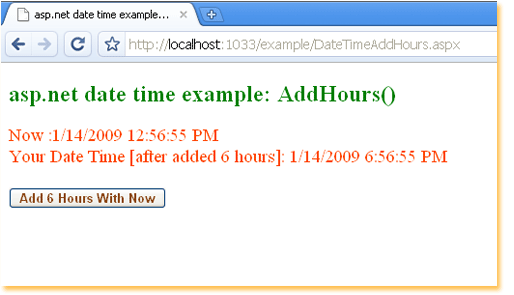### c# - How to add hours to DateTime

The following asp.net c# example code demonstrate us how can we add one or more hours to a DateTime object programmatically at run time. .Net framework's DateTime.AddHours() method return a new DateTime object that add the specified number of hours to the value of this instance.

DateTime.AddHours() method have a required parameter. This parameter name is 'value' and its data type is System.Double. This Double value represent a number of whole and fractional hours. This parameter value can be negative or positive. So, we can also subtract hours from a DateTime object if we pass a negative value as parameter.

DateTime.AddHours() method return a System.DateTime type object. The return DateTime object is the sum of DateTime instance and the number of hours passes by parameter. The fractional part of the 'value' parameter value is the fractional part of an hour. Such as Parameter value 5.5 is equivalent to 5 hours and 30 minutes.

This method does not change/modify the provided dateTime object instead it return a new DateTime object which is the result of this operation.
``````
<%@ Page Language="C#" %>

<!DOCTYPE html>

<script runat="server">
protected void Page_Load(object sender, System.EventArgs e) {
Label1.Text = "Now :" + DateTime.Now.ToString();
}
protected void Button1_Click(object sender, System.EventArgs e) {
DateTime now = DateTime.Now;
Label1.ForeColor = System.Drawing.Color.OrangeRed;
Label1.Text ="Now :" + now.ToString();
Label1.Text += "<br />Your Date Time [after added 6 hours]: " + modifiedDatetime.ToString();

}
</script>

<html xmlns="http://www.w3.org/1999/xhtml">
<body>
<form id="form1" runat="server">
<div>
<h2 style="color:Green">asp.net date time example: AddHours()</h2>
<asp:Label
ID="Label1"
runat="server"
Font-Size="Larger"
>
</asp:Label>
<br /><br />
<asp:Button
ID="Button1"
runat="server"
Font-Bold="true"